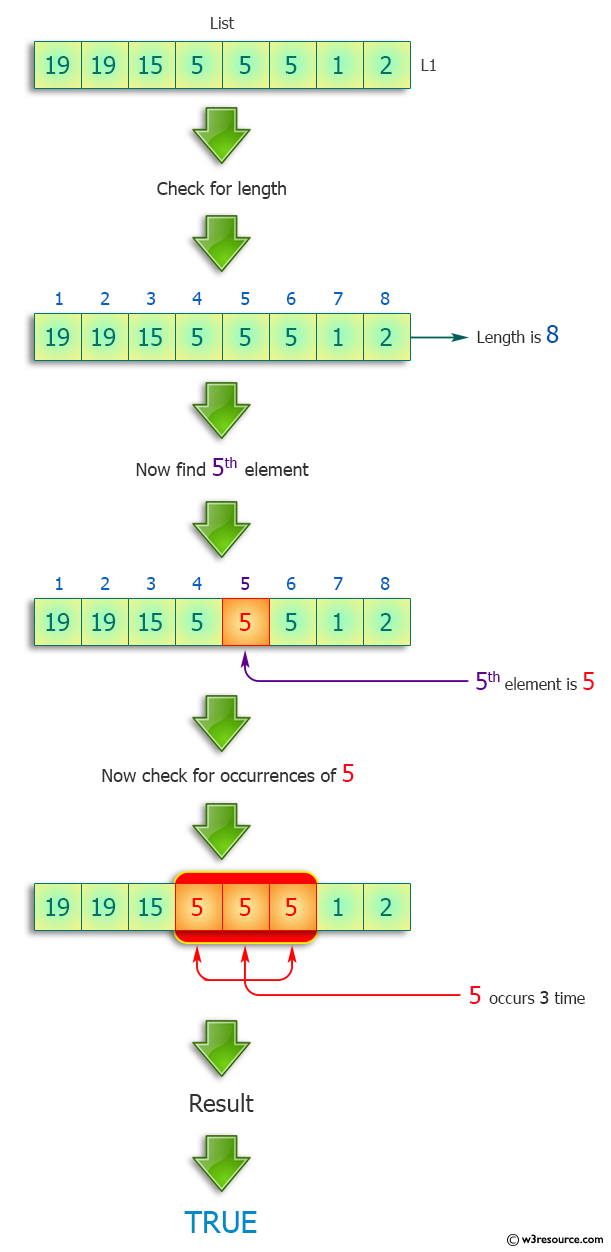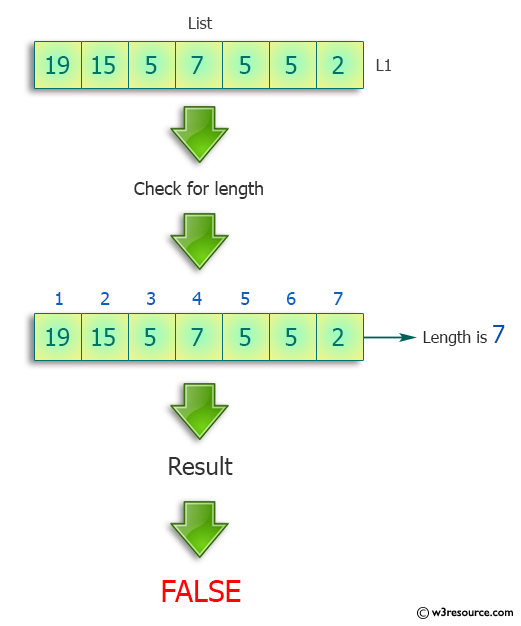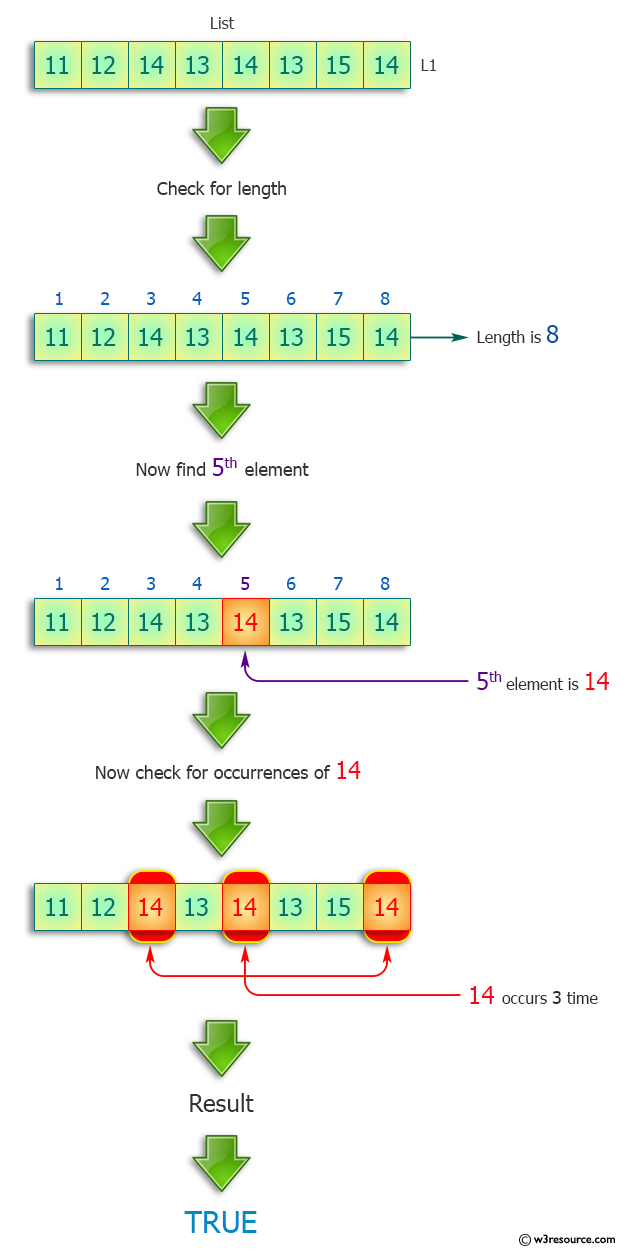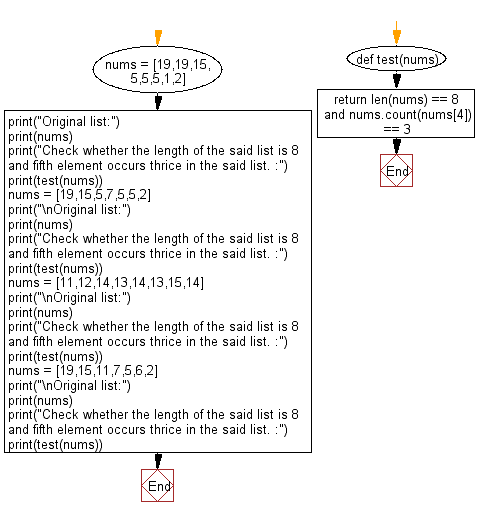﻿ Python: Check the length and the fifth element occurs twice in a list - w3resource# Python: Check the length and the fifth element occurs twice in a list

## Python Programming Puzzles: Exercise-2 with Solution

Write a Python program that accept a list of integers and check the length and the fifth element. Return true if the length of the list is 8 and fifth element occurs thrice in the said list.

```Input:
[19, 19, 15, 5, 5, 5, 1, 2]
Output:
True

Input:
[19, 15, 5, 7, 5, 5, 2]
Output:
False

Input:
[11, 12, 14, 13, 14, 13, 15, 14]
Output:
True

Input:
[19, 15, 11, 7, 5, 6, 2]
Output:
False
```

Pictorial Presentation:Sample Solution:

Python Code:

``````#License: https://bit.ly/3oLErEI

def test(nums):
return len(nums) == 8 and nums.count(nums) == 3

nums = [19,19,15,5,5,5,1,2]
print("Original list:")
print(nums)
print("Check whether the length of the said list is 8 and fifth element occurs thrice in the said list. :")
print(test(nums))
nums = [19,15,5,7,5,5,2]
print("\nOriginal list:")
print(nums)
print("Check whether the length of the said list is 8 and fifth element occurs thrice in the said list. :")
print(test(nums))
nums = [11,12,14,13,14,13,15,14]
print("\nOriginal list:")
print(nums)
print("Check whether the length of the said list is 8 and fifth element occurs thrice in the said list. :")
print(test(nums))
nums = [19,15,11,7,5,6,2]
print("\nOriginal list:")
print(nums)
print("Check whether the length of the said list is 8 and fifth element occurs thrice in the said list. :")
print(test(nums))
``````

Sample Output:

```Original list:
[19, 19, 15, 5, 5, 5, 1, 2]
Check whether the length of the said list is 8 and fifth element occurs thrice in the said list. :
True

Original list:
[19, 15, 5, 7, 5, 5, 2]
Check whether the length of the said list is 8 and fifth element occurs thrice in the said list. :
False

Original list:
[11, 12, 14, 13, 14, 13, 15, 14]
Check whether the length of the said list is 8 and fifth element occurs thrice in the said list. :
True

Original list:
[19, 15, 11, 7, 5, 6, 2]
Check whether the length of the said list is 8 and fifth element occurs thrice in the said list. :
False
```

Flowchart:## Visualize Python code execution:

The following tool visualize what the computer is doing step-by-step as it executes the said program:

Python Code Editor :

Have another way to solve this solution? Contribute your code (and comments) through Disqus.

What is the difficulty level of this exercise?

Test your Programming skills with w3resource's quiz.

﻿

## Python: Tips of the Day

Clamps num within the inclusive range specified by the boundary values x and y:

Example:

```def tips_clamp_num(num,x,y):
return max(min(num, max(x, y)), min(x, y))
print(tips_clamp_num(2, 4, 6))
print(tips_clamp_num(1, -1, -6))
```

Output:

```4
-1
```